### Home > CALC > Chapter 4 > Lesson 4.3.2 > Problem4-112

4-112.
1. Refer to the graph below of f ′(x), the derivative of some function f(x). Homework Help ✎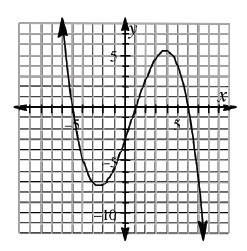1. Where is f(x) increasing? How can you tell?

2. Approximate the interval on which f(x) is concave up. Justify your conclusion with the graph.

3. Is f ′′(0) positive or negative? Explain how you know.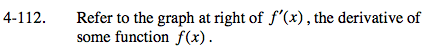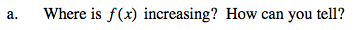Notice that this is the graph of f '(x), the slopes of f(x), and f(x) will increase where its slopes are positive.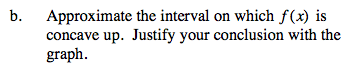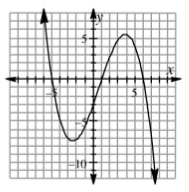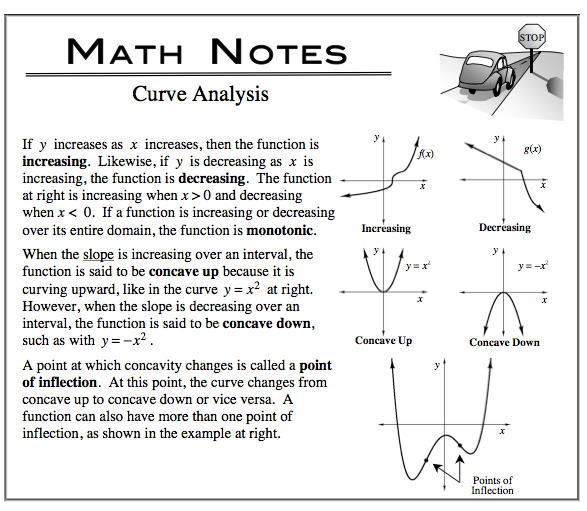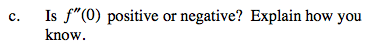f ''(x) represents the slopes of the given graph, f '(x). So look at the graph. Does it have a positive or a negative slope at x = 0?